Home
Hostname: page-component-55597f9d44-xbgml Total loading time: 0.41 Render date: 2022-08-18T14:08:57.606Z Has data issue: true Feature Flags: { "shouldUseShareProductTool": true, "shouldUseHypothesis": true, "isUnsiloEnabled": true, "useRatesEcommerce": false, "useNewApi": true } hasContentIssue true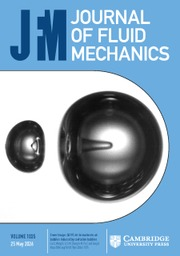Journal of Fluid Mechanics

## Abstract

A group of three multiscale inhomogeneous grids have been tested to generate different types of turbulent shear flows with different mean shear rate and turbulence intensity profiles. Cross hot-wire measurements were taken in a wind tunnel with Reynolds number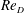$Re_{D}$ of 6000–20 000, based on the width of the vertical bars of the grid and the incoming flow velocity. The effect of local drag coefficient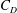$C_{D}$ on the mean velocity profile is discussed first, and then by modifying the vertical bars to obtain a uniform aspect ratio the mean velocity profile is shown to be predictable using the local blockage ratio profile. It is also shown that, at a streamwise location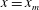$x=x_{m}$, the turbulence intensity profile along the vertical direction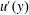$u^{\prime }(y)$ scales with the wake interaction length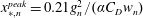$x_{\ast ,n}^{peak}=0.21g_{n}^{2}/(\unicode[STIX]{x1D6FC}C_{D}w_{n})$ ($\unicode[STIX]{x1D6FC}$ is a constant characterizing the incoming flow condition, and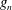$g_{n}$,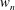$w_{n}$ are the gap and width of the vertical bars, respectively, at layer$n$) such that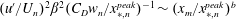$(u^{\prime }/U_{n})^{2}\unicode[STIX]{x1D6FD}^{2}(C_{D}w_{n}/x_{\ast ,n}^{peak})^{-1}\sim (x_{m}/x_{\ast ,n}^{peak})^{b}$, where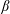$\unicode[STIX]{x1D6FD}$ is a constant determined by the free-stream turbulence level,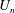$U_{n}$ is the local mean velocity and$b$ is a dimensionless power law constant. A general framework of grid design method based on these scalings is proposed and discussed. From the evolution of the shear stress coefficient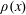$\unicode[STIX]{x1D70C}(x)$, integral length scale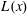$L(x)$ and the dissipation coefficient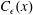$C_{\unicode[STIX]{x1D716}}(x)$, a simple turbulent kinetic energy model is proposed that describes the evolution of our grid generated turbulence field using one centreline measurement and one vertical profile of$u^{\prime }(y)$ at the beginning of the evolution. The results calculated from our model agree well with our measurements in the streamwise extent up to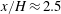$x/H\approx 2.5$, where$H$ is the height of the grid, suggesting that it might be possible to design some shear flows with desired mean velocity and turbulence intensity profiles by designing the geometry of a passive grid.

## JFM classification

Type
JFM Papers
Information
Journal of Fluid Mechanics , 10 August 2018 , pp. 788 - 820

## Access options

Get access to the full version of this content by using one of the access options below. (Log in options will check for institutional or personal access. Content may require purchase if you do not have access.)

## References

Bai, K., Meneveau, C. & Katz, J. 2012 Near-wake turbulent flow structure and mixing length downstream of a fractal tree. Boundary-Layer Meteorology 143 (2), 285308.CrossRefGoogle Scholar
Bearman, P. W. & Trueman, D. M. 1972 Investigation of flow around rectangular cylinders. Aeronaut. Q. 23 (AUG), 229237.Google Scholar
Bottin, S., Dauchot, O., Daviaud, F. & Manneville, P. 1998 Experimental evidence of streamwise vortices as finite amplitude solutions in transitional plane couette flow. Phys. Fluids 10 (10), 25972607.CrossRefGoogle Scholar
Cardesa, J. I., Nickels, T. B. & Dawson, J. R. 2012 2D PIV measurements in the near field of grid turbulence using stitched fields from multiple cameras. Exp. Fluids 52 (6), 16111627.CrossRefGoogle Scholar
Cekli, H. & van de Water, W. 2010 Tailoring turbulence with an active grid. Exp. Fluids 49 (2), 409416.CrossRefGoogle Scholar
Champagne, F. H., Harris, V. G. & Corrsin, S. 1970 Experiments on nearly homogeneous turbulent shear flow. J. Fluid Mech. 41 (01), 81139.CrossRefGoogle Scholar
Coles, D. 1965 Transition in circular couette flow. J. Fluid Mech. 21 (3), 385425.CrossRefGoogle Scholar
Comte-Bellot, G. & Corrsin, S. 1966 The use of a contraction to improve the isotropy of grid-generated turbulence. J. Fluid Mech. 25 (04), 657682.CrossRefGoogle Scholar
Comte-Bellot, G. & Corrsin, S. 1971 Simple eulerian time correlation of full- and narrow-band velocity signals in grid-generated, isotropic turbulence. J. Fluid Mech. 48 (02), 273337.CrossRefGoogle Scholar
Cornell, W. G. 1958 Losses in flow normal to plane screens. Trans. ASME 80 (4), 791799.Google Scholar
Corrsin, S. 1963 Turbulence: experimental methods. Handbuch der Physik 3, 524590.Google Scholar
Dunn, W. & Tavoularis, S. 2007 The use of curved screens for generating uniform shear at low Reynolds numbers. Exp. Fluids 42 (2), 281290.CrossRefGoogle Scholar
Elder, J. W. 1959 Steady flow through non-uniform gauzes of arbitrary shape. J. Fluid Mech. 5 (03), 355368.CrossRefGoogle Scholar
Gad-El-Hak, M. & Corrsin, S. 1974 Measurements of the nearly isotropic turbulence behind a uniform jet grid. J. Fluid Mech. 62 (01), 115143.CrossRefGoogle Scholar
Garg, S. & Warhaft, Z. 1998 On the small scale structure of simple shear flow. Phys. Fluids 10 (3), 662673.CrossRefGoogle Scholar
Gomes-Fernandes, R., Ganapathisubramani, B. & Vassilicos, J. C. 2012 Particle image velocimetry study of fractal-generated turbulence. J. Fluid Mech. 711, 306336.CrossRefGoogle Scholar
Harris, V. G., Graham, J. A. H. & Corrsin, S. 1977 Further experiments in nearly homogeneous turbulent shear flow. J. Fluid Mech. 81 (04), 657687.CrossRefGoogle Scholar
Hearst, R. J. & Ganapathisubramani, B. 2017 Tailoring incoming shear and turbulence profiles for lab-scale wind turbines. Wind Energy 20 (12), 20212035.CrossRefGoogle Scholar
Hearst, R. J. & Lavoie, P. 2014 Decay of turbulence generated by a square-fractal-element grid. J. Fluid Mech. 741, 567584.CrossRefGoogle Scholar
Hurst, D. & Vassilicos, J. C. 2007 Scalings and decay of fractal-generated turbulence. Phys. Fluids 19 (3), 035103.CrossRefGoogle Scholar
Isaza, J. C., Salazar, R. & Warhaft, Z. 2014 On grid-generated turbulence in the near- and far field regions. J. Fluid Mech. 753, 402426.CrossRefGoogle Scholar
Karnik, U. & Tavoularis, S. 1987 Generation and manipulation of uniform shear with the use of screens. Exp. Fluids 5 (4), 247254.CrossRefGoogle Scholar
Knebel, P., Kittel, A. & Peinke, J. 2011 Atmospheric wind field conditions generated by active grids. Exp. Fluids 51 (2), 471481.CrossRefGoogle Scholar
Laws, E. M. & Livesey, J. L. 1978 Flow through screens. Annu. Rev. Fluid Mech. 10 (1), 247266.CrossRefGoogle Scholar
Lawson, T. V. 1968 Methods of producing velocity profiles in wind tunnels. Atmos. Environ. 2 (1), 7376.CrossRefGoogle Scholar
Livesey, J. L. & Laws, E. M. 1973a Flow through non-uniform gauze screens. J. Fluid Mech. 59 (04), 737743.CrossRefGoogle Scholar
Livesey, J. L. & Laws, E. M. 1973b Simulation of velocity profiles by shaped gauze screens. AIAA J. 11 (2), 184188.CrossRefGoogle Scholar
Makita, H. 1991 Realization of a large-scale turbulence field in a small wind tunnel. Fluid Dyn. Res. 8 (1–4), 5364.Google Scholar
Mazellier, N. & Vassilicos, J. C. 2010 Turbulence without Richardson–Kolmogorov cascade. Phys. Fluids 22 (7), 075101,1–25.CrossRefGoogle Scholar
McCarthy, J. H. 1964 Steady flow past non-uniform wire grids. J. Fluid Mech. 19 (04), 491512.CrossRefGoogle Scholar
Mohamed, M. S. & Larue, J. C. 1990 The decay power law in grid-generated turbulence. J. Fluid Mech. 219, 195214.CrossRefGoogle Scholar
Mulhearn, P. J. & Luxton, R. E. 1975 The development of turbulence structure in a uniform shear flow. J. Fluid Mech. 68 (03), 577590.CrossRefGoogle Scholar
Mydlarski, L. & Warhaft, Z. 1996 On the onset of high-Reynolds-number grid-generated wind tunnel turbulence. J. Fluid Mech. 320, 331368.CrossRefGoogle Scholar
Nedić, J. & Tavoularis, S. 2016 Energy dissipation scaling in uniformly sheared turbulence. Phys. Rev. E 93 (3), 033115.Google ScholarPubMed
O’Neill, P. L., Nicolaides, D., Honnery, D. & Soria, J. 2004 Autocorrelation functions and the determination of integral length with reference to experimental and numerical data. In 15th Australasian Fluid Mechanics Conference. The University of Sydney.Google Scholar
Owen, P. R. & Zienkiewicz, H. K. 1957 The production of uniform shear flow in a wind tunnel. J. Fluid Mech. 2 (06), 521531.CrossRefGoogle Scholar
Richards, H. K. & Morton, J. B. 1976 Experimental investigation of turbulent shear flow with quadratic mean-velocity profiles. J. Fluid Mech. 73 (01), 165188.CrossRefGoogle Scholar
Roach, P. E. 1987 The generation of nearly isotropic turbulence by means of grids. Int. J. Heat Fluid Fl. 8 (2), 8292.CrossRefGoogle Scholar
Rohr, J. J., Itsweire, E. C., Helland, K. N. & Van Atta, C. W. 1988 An investigation of the growth of turbulence in a uniform-mean-shear flow. J. Fluid Mech. 187, 133.CrossRefGoogle Scholar
Rose, W. G. 1966 Results of an attempt to generate a homogeneous turbulent shear flow. J. Fluid Mech. 25 (01), 97120.CrossRefGoogle Scholar
Rose, W. G. 1970 Interaction of grid turbulence with a uniform mean shear. J. Fluid Mech. 44 (04), 767779.CrossRefGoogle Scholar
Schumacher, J. R. G. 2001 Derivative moments in stationary homogeneous shear turbulence. J. Fluid Mech. 441, 109118.CrossRefGoogle Scholar
Seoud, R. E. & Vassilicos, J. C. 2007 Dissipation and decay of fractal-generated turbulence. Phys. Fluids 19 (10), 105108.CrossRefGoogle Scholar
Shen, X. & Warhaft, Z. 2000 The anisotropy of the small scale structure in high Reynolds number (Re ∼ 1000) turbulent shear flow. Phys. Fluids 12 (11), 29762989.CrossRefGoogle Scholar
de Souza, F. A., Nguyen, V. D. & Tavoularis, S. 1995 The structure of highly sheared turbulence. J. Fluid Mech. 303, 155167.CrossRefGoogle Scholar
Tavoularis, S. & Corrsin, S. 1981 Experiments in nearly homogenous turbulent shear flow with a uniform mean temperature gradient. Part 1. J. Fluid Mech. 104, 311347.CrossRefGoogle Scholar
Tavoularis, S. & Karnik, U. 1989 Further experiments on the evolution of turbulent stresses and scales in uniformly sheared turbulence. J. Fluid Mech. 204, 457478.CrossRefGoogle Scholar
Taylor, G. I., Batchelor, G. K., Dryden, H. L. & Schubauer, G. B. 1949 The effect of wire gauze on small disturbances in a uniform stream. Q. J. Mech. Appl. Math. 2 (1), 129.CrossRefGoogle Scholar
Tennekes, H. & Lumley, J. L. 1972 A First Course in Turbulence. MIT Press.Google Scholar
Thormann, A. & Meneveau, C. 2015 Decaying turbulence in the presence of a shearless uniform kinetic energy gradient. J. Turbul. 16 (5), 442459.CrossRefGoogle Scholar
Tillmark, N. & Alfredsson, P. H. 1992 Experiments on transition in plane couette flow. J. Fluid Mech. 235, 89102.CrossRefGoogle Scholar
Valente, P. C. & Vassilicos, J. C. 2011 The decay of turbulence generated by a class of multiscale grids. J. Fluid Mech. 687, 300340.CrossRefGoogle Scholar
Vanderwel, C. & Tavoularis, S. 2014 Measurements of turbulent diffusion in uniformly sheared flow. J. Fluid Mech. 754, 488514.CrossRefGoogle Scholar
Wilson, D. K.1998 A new model for turbulence spectra and correlations based on Meijer’s G-functions. Technical Note ARL-TN-104. Army Research Laboratory.Google Scholar
Zhu, W., van Hout, R., Luznik, L., Kang, H. S., Katz, J. & Meneveau, C. 2006 A comparison of PIV measurements of canopy turbulence performed in the field and in a wind tunnel model. Exp. Fluids 41 (2), 309318.CrossRefGoogle Scholar
1
Cited by

# Save article to Kindle

Note you can select to save to either the @free.kindle.com or @kindle.com variations. ‘@free.kindle.com’ emails are free but can only be saved to your device when it is connected to wi-fi. ‘@kindle.com’ emails can be delivered even when you are not connected to wi-fi, but note that service fees apply.

Find out more about the Kindle Personal Document Service.

Weakly sheared turbulent flows generated by multiscale inhomogeneous grids
Available formats
×

# Save article to Dropbox

To save this article to your Dropbox account, please select one or more formats and confirm that you agree to abide by our usage policies. If this is the first time you used this feature, you will be asked to authorise Cambridge Core to connect with your Dropbox account. Find out more about saving content to Dropbox.

Weakly sheared turbulent flows generated by multiscale inhomogeneous grids
Available formats
×

# Save article to Google Drive

To save this article to your Google Drive account, please select one or more formats and confirm that you agree to abide by our usage policies. If this is the first time you used this feature, you will be asked to authorise Cambridge Core to connect with your Google Drive account. Find out more about saving content to Google Drive.

Weakly sheared turbulent flows generated by multiscale inhomogeneous grids
Available formats
×
×